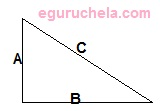# Triangle Inequality Solver

Enter Any 2 Sides

 Side1 : Side2 :

 Side3 =

The triangle is said to be in inequality state, when the sum of the two sides of a triangle is greater than that of the other side. The sum of the lengths of any 2 sides of a triangle must exceed the length of the third side.Triangle inequality states that as

A + B > C

B + C > A

A + C > B

where,

A, B and C are the side lengths